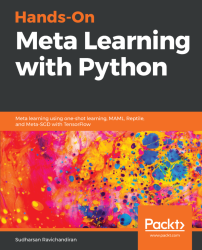•#### Hands-On Meta Learning with Python#### Overview of this book

Meta learning is an exciting research trend in machine learning, which enables a model to understand the learning process. Unlike other ML paradigms, with meta learning you can learn from small datasets faster. Hands-On Meta Learning with Python starts by explaining the fundamentals of meta learning and helps you understand the concept of learning to learn. You will delve into various one-shot learning algorithms, like siamese, prototypical, relation and memory-augmented networks by implementing them in TensorFlow and Keras. As you make your way through the book, you will dive into state-of-the-art meta learning algorithms such as MAML, Reptile, and CAML. You will then explore how to learn quickly with Meta-SGD and discover how you can perform unsupervised learning using meta learning with CACTUs. In the concluding chapters, you will work through recent trends in meta learning such as adversarial meta learning, task agnostic meta learning, and meta imitation learning. By the end of this book, you will be familiar with state-of-the-art meta learning algorithms and able to enable human-like cognition for your machine learning models.
Title PageDedicationContributorsPrefaceFree Chapter
Introduction to Meta LearningFace and Audio Recognition Using Siamese NetworksPrototypical Networks and Their VariantsRelation and Matching Networks Using TensorFlowMemory-Augmented Neural NetworksMAML and Its VariantsMeta-SGD and ReptileGradient Agreement as an Optimization ObjectiveAssessmentsOther Books You May EnjoyIndex## Building relation networks using TensorFlow

The relation function is pretty simple, right? We will understand relation networks better by implementing one in TensorFlow.

You can also check the code available as a Jupyter Notebook with an explanation here: https://github.com/sudharsan13296/Hands-On-Meta-Learning-With-Python/blob/master/04.%20Relation%20and%20Matching%20Networks%20Using%20Tensorflow/4.5%20Building%20Relation%20Network%20Using%20Tensorflow.ipynb.

First, we import all of the required libraries:

```import tensorflow as tf
import numpy as np```

We will randomly generate our data points. Let's say we have two classes in our dataset; we will randomly generate some 1,000 data points for each of these classes:

```classA = np.random.rand(1000,18)
ClassB = np.random.rand(1000,18)```

We create our dataset by combining both of these classes:

`data = np.vstack([classA, ClassB])`

Now, we set the labels; we assign the `1`label for `classA` and the `0`label for `classB`:

`label = np.vstack([np.ones((len(classA),1)),np.zeros...`